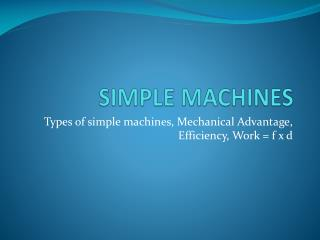DownloadDownload PresentationSIMPLE MACHINES

# SIMPLE MACHINES

Download Presentation## SIMPLE MACHINES

- - - - - - - - - - - - - - - - - - - - - - - - - - - E N D - - - - - - - - - - - - - - - - - - - - - - - - - - -
##### Presentation Transcript

1. SIMPLE MACHINES Types of simple machines, Mechanical Advantage, Efficiency, Work = f x d

2. Simple Machines There are six types of simple machines: • Pulleys • Levers • Inclined Plane • Wedge • Screw • Wheel and Axle

3. Pulleys 1. A fixed pulley does not change the force applied, but changes the direction of the force that needs to be applied. F = 100N and d = 10cm. Its MA=1.

4. Pulleys 2. In this setup the force has decreased, but you had to apply the force through a longer distance. F = 50N and d = 20cm Its Mechanical Advantage =2

5. Pulleys 3. In this setup the force has decreased more, but distance has increased. F = 33.5N and d = 30cm Its Mechanical Advantage =3

6. Pulleys 4. In this setup the force has decreased more but the distance has increases a lot more. F = 25N and d = 40cm Its Mechanical Advantage = 4

7. Machines Work is defined as the force applied through a given displacement. (W = f x d) If you stand holding a bucket, you may get tired, but actually you do no work. Machines help decrease the force we have to apply, but increase the distance…. When we apply less force, we think we are doing less work!!!

8. Mechanical Advantage The smaller the force that needs to be applied, the bigger the mechanical advantage. Mechanical advantage = output distance/ Input distance

9. Efficiency: • Efficiency is defined as the effectiveness of a given machine. It can be measured by comparing its output work to its input work. Workoutput Efficiency =______________ x 100 Work input • The more machines you use, the smaller the efficiency (because in real life, there is more loss to friction in complex machines).

10. MA and Efficiency The more the MA, the smaller the efficiency as more energy is lost to friction. ( - We have to do the actual work and also more to make up for the frictional work.) (- We do not realize it as we confuse work with force. We think less force => less work)